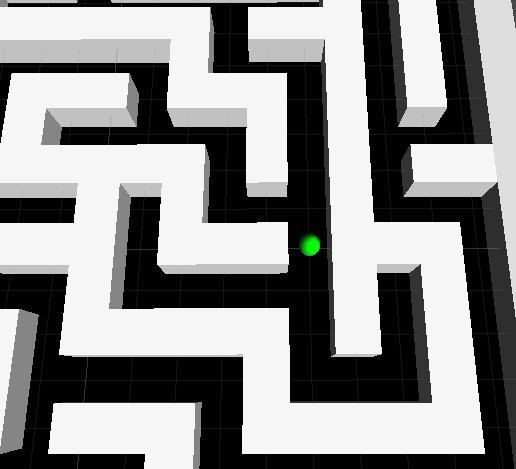# Limited player walk in the center of road in labyrinthi want to limited player always walk in the center of road,when right has no path,it can’t move to right , not like thati can’t make the player scale equal width of road.

how to fix it,i need help.

A simple solution is to stretch the player collider: you don’t have to modify its scale, only the collider or CharacterController radius.

The other solution is to snap the player position to a grid, but this alternative may complicate a lot the movement script: you should calculate the target grid position according to the controls, verify whether it’s not blocked by walls, then move the player gradually to this position. Post your current movement script, and maybe we can suggest the necessary changes.

EDITED: This is a (highly) modified version of your script that walks in a grid pattern like said above. The grid is aligned to the axes X and Z, and the initial position of your character is assumed to be the grid “origin” - all other grid positions are at multiples of gridSize from this point, measured in the X and/or Z axes. It’s advisable to choose an integer value for gridSize (or multiple of 0.5), since most non-integer values don’t have exact representation in floating point format - this may cause precision errors after a lot of walking.

The Update function has two parts: if a control button is pressed, the script calculates the new target position and starts moving. The controls are ignored until the character reaches the target position, or the time maxMoveTime has elapsed (this avoids the character getting stuck in certain cases, like diagonal movement).

``````#pragma strict

var gf_moveSpeed : float = 6;
var gf_gravity : float = 20;
var gf_jumpSpeed : float = 6;
var gridSize : float = 2.0; // space between grid points
var maxMoveTime : float = 0.5; // max time to reach target position
public var isDead : boolean = false;

private var lc_controller : CharacterController;
private var lc_myTransform : Transform;
private var targetPos: Vector3; // target position
private var velY : float = 0; // vertical velocity
private var moving: float = 0; // count time while moving to target pos
private var isThirdPerson : boolean = true;

function Start () {
lc_controller = GetComponent(CharacterController);
lc_myTransform = transform;
if (!lc_controller){
Debug.Log("Can not get CharacterController");
}
targetPos = lc_myTransform.position;
}

function Update () {

var delta = Vector3.zero; // delta is distance to move this frame
if (moving <= 0){ // if not moving...
var curTarget = targetPos;
var dirZ = Input.GetAxis("Vertical");
if (dirZ > 0){ //forward pressed?
// yes: set new target position IF no obstacle ahead
if (!Physics.Raycast(curTarget, Vector3.forward, gridSize)) targetPos.z += gridSize;
}
else
if (dirZ < 0){  // back pressed?
// yes: set new target position IF no obstacle behind
if (!Physics.Raycast(curTarget, Vector3.back, gridSize)) targetPos.z -= gridSize;
}
var dirX = Input.GetAxis("Horizontal");
if (dirX > 0){ // right pressed?
// yes: set new target position IF no obstacle at right
if (!Physics.Raycast(curTarget, Vector3.right, gridSize)) targetPos.x += gridSize;
}
else
if (dirX < 0){ // left pressed?
// yes: set new target position IF no obstacle at left
if (!Physics.Raycast(curTarget, Vector3.left, gridSize)) targetPos.x -= gridSize;
}
if (targetPos != curTarget){
moving = maxMoveTime;
}
} else {
// is moving: calculate distance to target position:
delta = targetPos - lc_myTransform.position;
delta.y = 0; // ignore vertical distance
// calculate max distance to walk this frame at moveSpeed:
var maxDelta = gf_moveSpeed * Time.deltaTime;
if (delta.magnitude < maxDelta){ // will reach target this frame:
moving = 0; // no movement next frame
} else {
// limit movement according to moveSpeed
delta = Vector3.ClampMagnitude(delta, maxDelta);
// count time to avoid getting stuck at low obstacles
moving -= Time.deltaTime;
}
}
if (lc_controller.isGrounded){ // if character grounded...
velY = 0; // its vertical velocity is zero
}
// apply gravity acceleration to vertical velocity
velY -= gf_gravity * Time.deltaTime;
// add vertical movement to variable delta:
delta.y = velY * Time.deltaTime;
// move character
lc_controller.Move(delta);
}
``````